Thank you for visiting nature.com. You are using a browser version with limited support for CSS. To obtain the best experience, we recommend you use a more up to date browser (or turn off compatibility mode in Internet Explorer). In the meantime, to ensure continued support, we are displaying the site without styles and JavaScript.

# Measurement of geometric dephasing using a superconducting qubit

## Abstract

A quantum system interacting with its environment is subject to dephasing, which ultimately destroys the information it holds. Here we use a superconducting qubit to experimentally show that this dephasing has both dynamic and geometric origins. It is found that geometric dephasing, which is present even in the adiabatic limit and when no geometric phase is acquired, can either reduce or restore coherence depending on the orientation of the path the qubit traces out in its projective Hilbert space. It accompanies the evolution of any system in Hilbert space subjected to noise.

## Introduction

The information stored in a quantum bit is ultimately lost when the interaction with its environment causes randomization of its quantum phase in a process known as dephasing1. Part of this dephasing is of geometric origin2 and is related to a type of geometric phase known as Berry phase3,4, which is accumulated when a quantum system is adiabatically steered along a closed contour in the parameter space of its Hamiltonian. This phase underlines the dynamics and thermodynamics of a broad spectrum of quantum systems as measured in spins5,6, systems dominated by spin–orbit coupling7,8,9, experiments exhibiting Aharonov–Bohm phases10, as well as atomic11,12 and optical set-ups13,14.

The geometric phase roots in the structure of Hilbert space and is—unlike the dynamic phase—not related to the duration of a quantum process. When it comes to quantum dissipative systems, ubiquitous in our physical world, the nature of the geometric phase may be put to question: noise may screen out geometric effects and the condition for adiabaticity is not self-evident. The former effect is frequently modelled by adding non-geometric dissipative rates to the equations of motion for the density matrix, see for example, ref. 15. The latter concern is alleviated in ref. 16: naively one may expect that adiabaticity implies that the rate of change involved is smaller than the excitation gap. It turns out though that a gapless spectrum, which may be the result of coupling the system to a dissipative reservoir, does not necessarily annul the Berry phase3,4. Moreover, dephasing and level broadening due to classical low-frequency noise randomizing the Berry phase are addressed in refs 17, 18; corrections to the Berry phase, due to both slow, classical noise and high-frequency quantum noise have been discussed in ref. 2. In that work, the concept of geometric dephasing has been introduced. Indeed, decoherence in quantum systems stems not only from the stochastic evolution of the dynamic phase of the system’s wave function (dynamic dephasing), but also from geometric effects.

In this article, we experimentally confirm the existence of geometric dephasing using a superconducting quantum system19 exposed to artificial noise. We find that geometric dephasing can either reduce or restore coherence depending on the orientation of the path a qubit traces out in its projective Hilbert space. This asymmetric decoherence mechanism is expected to play a role in numerous stochastic systems exhibiting geometric phases20,21.

## Results

### Experimental background

Our experiment complements earlier ones on orientation-independent dephasing induced by non-adiabatic corrections to the Berry phase22,23, and on the effect of classical noise24 and a quantum bath25 on the geometric phase.

Below, we show that the state (Bloch) vector of a qubit evolving adiabatically in the presence of Gaussian noise contains a suppression factor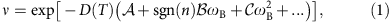where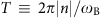is the duration of the time evolution,the oriented number of loops of the qubit in its projective Hilbert space (equal to the number of loops performed by the magnetic field for adiabatic evolution), ωB>0 the precession frequency and 2π/ωB the duration of a single loop. The function D(T) describes the spectral properties of the noise. The first term in equation (1) is independent of ωB and represents dynamic dephasing. The second term, proportional to sgn(n)ωB, represents geometric dephasing. The third term goes as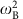and stands for non-geometric non-adiabatic dephasing. It is especially noteworthy that the second term can either increase or decrease the total dephasing, depending on the sign of n. This makes the geometric nature of this term explicit. The contribution to dephasing stemming from the first term is independent of the sign of n and is therefore not geometric. Likewise, the third term does not describe geometric dephasing. However, it leads to fluctuations in the Berry phase18 as observed in refs 22, 23.

In our experiments, a superconducting qubit (Supplementary Methods and Supplementary Fig. 1) is subject to resonant driving with slowly modulated amplitude and phase. In a frame corotating with the drive, the system can be modelled as a qubit in a slowly varying magnetic field—a paradigmatic system to observe the Berry phase5,22,26. To study geometric dephasing, we add artificial noise to the drive, mimicking noise in the magnetic field. Our analysis shows explicitly that the decay of a pure quantum state into a mixed state includes a term that is exponential in n, the number of oriented loops: the dephasing is asymmetric in the direction of the loops, as schematically represented in Fig. 1. The coherence (that is, the length of the Bloch vector) of the qubit recorded in a Ramsey-type interferometric experiment illustrates this phenomenon. As shown in Fig. 2a, a cyclic change of the effective magnetic field in clockwise (C++) or counter-clockwise (C−−) direction leads either to a decrease or an increase in coherence when compared with a static but still fluctuating magnetic field, as discussed in detail below.

The dynamics of the system in a frame rotating at the frequency ωd of the drive are described by the Hamiltonian (=1)26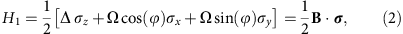where σ=(σx, σy, σz) are the Pauli matrices, Δ≡ω01ωd the detuning in frequency between drive and qubit transition, Ω(t) the amplitude andthe phase of the drive and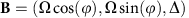the effective magnetic field in units of angular frequency. We let the magnetic field form an angle θ=arctan Ω/Δ with the Δ axis and have it precess about this axis at a rate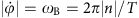. After a time T, the magnetic field has traced out n loops and enclosed a solid angle A=2(1−cosθ) as seen from B=0. If ωB is small enough, that is, if the evolution is adiabatic, the qubit’s state vector acquires a geometric phase A/2 (ref. 4). We induce fluctuations δΩ in the effective magnetic field in radial direction, which in our set-up correspond to amplitude noise in the signal driving the qubit, so that Ω(t)=Ω0+δΩ(t) and as a consequence θ(t)=θ0+δθ(t). Applying a transformation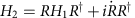with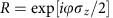(see for example, ref. 27) to equation (2) results in the HamiltonianFrom equation (3), it follows that in a Ramsey experiment, in which the effective magnetic field performs n oriented loops in the time interval [0, T], the eigenstates of the qubit acquire a total relative phase γ([0, T], n), where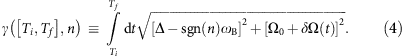We make sure that the conditionsand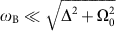are met, so that the qubit evolves adiabatically (also see Methods section). A second-order Taylor expansion of equation (4) in the two small parameters δΩ and ωB yieldsIn this expansion, where θ0 ≡ arctanΩ0/Δ, we have dropped two second-order terms which are non-consequential for our analysis: one(non-adiabatic corrections) and one δΩ2 (next-order correction to the dynamical phase and to the dynamical dephasing). The first two terms in equation (5) give rise to, the sum of dynamic and geometric phase in the absence of noise. The last two terms lead to dephasing, as seen by computing the variance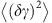of the phase in equation (5),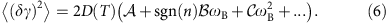Thus, we recover the suppression factor from equation (1) describing the length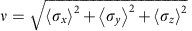of the state vector of the qubit. The function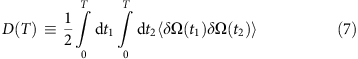is the integrated time-correlator of the noise. The functions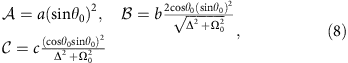appear in the three first terms in equation (6), which represent dynamic dephasing, geometric dephasing and non-geometric non-adiabatic corrections, respectively.

In equation (8) we have introduced dimensionless decoherence factors a, b, c (all equal to one in the Ramsey experiment considered) to accommodate different types of experiments, as detailed below. In particular, we use spin echo techniques to observe geometric dephasing while eliminating the dynamic phase and enhancing the qubit coherence time.

### Protocols for measuring dephasing

Two protocols leading to different decoherence factors a, b, c are considered. The first one is a complete echo, in which the orientation of the loop the magnetic field traverses is identical in both halves. In this protocol (named ‘protocol P’, as in ‘preserved’), the phase acquired by the qubit is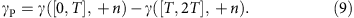In the second protocol, the orientation of the loop is reversed in the second half of the echo sequence. We therefore call it ‘protocol R’ (for ‘reversed’). The accumulated phase is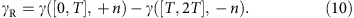To illustrate the protocols, schematics of the pulse sequences are shown in Fig. 3.

In our experiment, phase and coherence of the qubit have been recorded as a function of the solid angle enclosed by the effective magnetic field B. The detuning of the off-resonant pulses is Δ/2π=−35 MHz. The fluctuations δΩ applied to B conform to an Ornstein–Uhlenbeck process with correlation time 1/Γ=10 MHz, intensity σ2 and normalized noise amplitude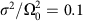. Intrinsic dephasing due to a finite decoherence rate of the qubit causes the coherence to drop to about 0.7 at the end of the pulse sequence. This effect is calibrated out23. The correlation function of the noise process is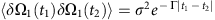, and the integrated time-correlator from equation (7) is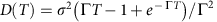. Since the noise δΩ(t) is artificial, we may control its time correlations, in particular those between the first time window, 0<t<T and the second time window, T<t<2T. For convenience, we define δΩ1(t) ≡ δΩ(t) and δΩ2(t) ≡ δΩ(t+T).

### Geometric and dynamic dephasing

A spin echo sequence with noise in the first window only, that is, δΩ2(t)≡0, allows us to measure the dephasing the qubit experiences during a Ramsey experiment. There is geometric dephasing in both protocols (b≠0 in Fig. 2a,c,e) which, depending on the sign of n, either increases or reduces the total dephasing. Interestingly, in protocol P geometric dephasing is present although the Berry phase (not shown) is eliminated along with the dynamic phase. This phenomenon can be explained by computing the variance of the phases in equations (9) and (10), from which the decoherence factors a=1, b=1 follow. In our experiment, a fit to the data yields a=1.14±0.02 for protocol P and a=1.07±0.02 for protocol R (Table 1), in good agreement with computations. The observed geometric dephasing (b=1.46±0.05 for protocol P and 1.58±0.12 for protocol R) is somewhat larger than predicted. The procedure used to extract a and b from fits to the data is described in the Methods section. The measured phases in protocol R agree with the prediction for a weakly anharmonic multi-level system28, with and without applied noise. However, when the noise causes the coherence to drop below ≈0.2, the phase can no longer be determined reliably and the measured phase deviates from theory.

Dynamic dephasing is more pronounced if the sequence is longer (T=160 ns in panel e, versus T=100 ns in panel c), but geometric dephasing is always present. The time-dependence in equation (1) describes the experimental data in Fig. 2 well, with a=1.12±0.03 and b=1.45±0.15 (for T=160 ns) and a=1.14±0.02 and b=1.58±0.12 (for T=100 ns). Examining the three contributions to dephasing appearing in the coherence suppression factor in equation (1) as a function of T (Fig. 2f), we see that the dynamic contribution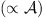grows with T, while the geometric contribution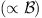saturates in the limit of short correlation times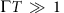: it does not vanish in the adiabatic limit T→∞. The non-geometric non-adiabatic contribution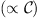vanishes in this limit. This holds for a general noise process (see Supplementary Methods).

While the experiment with noise in the first half of the spin echo only is adequate to measure geometric dephasing, a more physically relevant scenario is to consider noise appearing in both halves of the spin echo.

For uncorrelated noise, that is, when the correlation time of the noise is shorter than the timescale of the spin echo (as for example, for white noise29,30), we have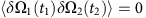. In addition, the spectral power of the noise is kept constant,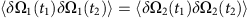. In this case, we measure geometric dephasing in the complete spin echo only (protocol P, Fig. 2b and Table 1). In protocol R, there is only dynamic dephasing (Fig. 2d, see Methods section for details).

For perfectly correlated noise δΩ1(t)=δΩ2(t), which corresponds to fluctuations slower than typical spin echo time scales as typical for 1/f-noise, neither dynamic nor geometric dephasing is expected. Non-adiabatic contributions (quantified by c) only play a role in protocol R, where c=4 is expected and c=2.25±0.25 is found from a fit to data (not shown). In protocol P, all fluctuations cancel out (a=b=c=0 in theory) and a fit gives c=0.24±0.54. The effect of correlated noise has been studied in refs 18, 22, 23.

Finally, we have also considered anticorrelated noise (δΩ1(t)=−δΩ2(t)) which maximizes both dynamic and geometric dephasing (Table 1) in accordance with expectations. As with uncorrelated noise, geometric dephasing is only present in protocol P.

Although in this experiment we consider geometric dephasing for closed loops, this effect may be detected even for open trajectories in parameter space: In any interferometric set-up, interference fringes originating from the non-unity overlap between the initial reference state and the final state after a non-cyclic evolution will exhibit dephasing in the form of a decaying envelope. Geometric dephasing will reveal itself as a rate of decay which depends on the direction, that is, the sign of n.

Given the broad spectrum of systems whose dynamics involves geometric phases, the presence of geometric dephasing is expected to be commonplace. While this additional contribution to decoherence is typically small, it can be of relevance for high-fidelity quantum operations and decoupling pulse techniques. For example, residual coupling to spurious modes will cause the system of interest to precess and induce dephasing with both dynamical and geometric contributions. Moreover, as shown here, geometric dephasing is present even when no geometric phase is acquired. One resulting intriguing question concerns the effect of geometric dephasing on the braiding phase of topological quasiparticles, when the separation of the relevant particles is within reach of stochastic fluctuations of the braiding path.

## Methods

The adiabaticity parameter is defined according to ref. 31. Given a Hamiltonian H(t), we can define an instantaneous (adiabatic) basis such that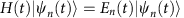. Writing D(t) for the transformation from a fixed basis (given for example, by H(0)) to the instantaneous basis, the Hamiltonian in the instantaneous basis is, with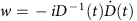. In the adiabatic case, w vanishes. The adiabatic parameter is defined as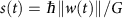, where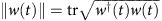is the trace norm of w(t) and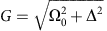is the energy gap in the spectrum of H(0). Evolution is adiabatic if.

The off-resonant pulses are shaped such that s is constant over time and independent of the solid angle when the drive Ω is increased or decreased (that is, at beginning and end of the pulses). For larger solid angles, the pulses need to be longer to keep s constant across solid angles. When the effective magnetic field precesses (central part of the pulses), s varies from solid angle to solid angle because ωB is kept constant. The data in Fig. 4 shows that s(t) is always smaller than 0.28 and thus adiabaticity is maintained during the whole off-resonant pulse sequence, even when noise is applied. As expected, s is smaller for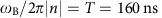than for T=100 ns.

Going to the extreme adiabatic limit s→0 is not desirable. Although geometric dephasing is still present in this limit, it cannot be resolved experimentally because dynamic dephasing increases linearly with time (see Supplementary Methods).

### Fitting the measured coherences

This section describes the fit models used to extract the parameters a, b and c quantifying dynamic and geometric dephasing.

In the fitting procedure, in a first step the effective normalized noise amplitude is found by fitting the function given in equation (1) describing the coherence ν to the data from protocol R with noise in the first window and dynamic phase only, assuming a=1, b=0, c=0 and with the normalized noise amplitude as the only fit parameter. In this way, a normalized noise amplitude ofwas determined, which is slightly smaller than the set value 0.1.

In a second step, all data from protocol R are fitted simultaneously for coefficients a and b of the functions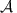and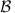. Where the theory predicts b=0, we do not wish to constrain the fitting function (and, by extension, limit the model) by setting b=0. Rather, we use a fitting function with a separate, primed variable, which ideally the fit then shows to be zero. We note that using the same variable b as in the other fits, where b≠0 is expected, is not possible, since all data is fitted simultaneously. As an example, consider protocol R with anticorrelated noise and C−+, where according to Table 2 no geometric dephasing (b=0) is expected: a fitting function with bb′ is used and the fit ideally produces b′=0. Similarly, for protocol R, dynamic phase, a variable b′′ is introduced to avoid unwanted interference with b′, as using a single parameter would prevent b′ from taking values other than zero in the fits to protocol R, C+− and C−+ , where b′=0 is expected. Finally, the proportionality factors of a and b in the fitting functions are fixed across the measurements. For instance, protocol R with anticorrelated noise uses 4a and protocol R, and with uncorrelated noise uses 2a. The data from protocol P is fitted similarly.

In a third step, the parameter c is only fitted for protocol R, correlated noise, C±, where a and b vanish. This is the only measurement where it is relevant.

Fourth, the fitting parameters b′ and b′′ are set to zero in the fit we call ‘constrained’. When dropping this constraint, the fitted values for a and b increase slightly (by maximally 6%, see Table 3); in turn, b′ and b′′ become negative. Simply put, the ‘unconstrained’ model (where b′ and b′′ are free parameters) trades-off some fitting parameters against each other to obtain the best fit. If theory was in perfect agreement with experimental data (no noise, no systematic errors), this trade-off would not be possible. However, owing to experimental imperfections the fit produces an unphysical result, namely ‘negative’ dynamic dephasing (b′, b′′<0). To avoid this effect, we have opted for presenting the constrained model in the main text. Comparing the parameter estimates in Table 3, it becomes apparent that both models yield similar parameter estimates. In addition, as discussed in the Supplementary Methods and in Supplementary Fig. 2, both models (constrained and unconstrained) have empirical support.

How to cite this article: Berger, S. et al. Measurement of geometric dephasing using a superconducting qubit. Nat. Commun. 6:8757 doi: 10.1038/ncomms9757 (2015).

## References

1. Schlosshauer, M. Decoherence and the Quantum-to-Classical Transition Springer (2007).

2. Whitney, R. S., Makhlin, Y., Shnirman, A. & Gefen, Y. Geometric nature of the environment-induced Berry phase and geometric dephasing. Phys. Rev. Lett. 94, 070407 (2005).

3. Pancharatnam, S. Generalized theory of interference and its applitactions. Proc. Indian Acad. Sci. A44, 247–262 (1956).

4. Berry, M. V. Quantal phase factors accompanying adiabatic changes. Proc. R. Soc. Lond. A392, 45–57 (1984).

5. Bitter, T. & Dubbers, D. Manifestation of Berry topological phase in neutron spin rotation. Phys. Rev. Lett. 59, 251–254 (1987).

6. Suter, D., Chingas, G. C., Harris, R. A. & Pines, A. Berry’s phase in magnetic resonance. Mol. Phys. 61, 1327–1340 (1987).

7. Meir, Y., Gefen, Y. & Entin-Wohlman, O. Universal effects of spin-orbit scattering in mesoscopic systems. Phys. Rev. Lett. 63, 798–800 (1989).

8. Loss, D., Goldbart, P. & Balatsky, A. V. Berry’s phase and persistent charge and spin currents in textured mesoscopic rings. Phys. Rev. Lett. 65, 1655–1658 (1990).

9. Nagasawa, F., Frustaglia, D., Saarikoski, H., Richter, K. & Nitta, J. Control of the spin geometric phase in semiconductor quantum rings. Nat. Commun. 4, 2526 (2013).

10. Chambers, R. G. Shift of an electron interference pattern by enclosed magnetic flux. Phys. Rev. Lett. 5, 3–5 (1960).

11. Atala, M. et al. Direct measurement of the Zak phase in topological Bloch bands. Nat. Phys. 9, 795–800 (2013).

12. Jotzu, G. et al. Experimental realization of the topological Haldane model with ultracold fermions. Nature 515, 237–240 (2014).

13. Tomita, A. & Chiao, R. Y. Observation of Berry topological phase by use of an optical fiber. Phys. Rev. Lett. 57, 937–940 (1986).

14. Loredo, J., Broome, M., Smith, D. & White, A. Observation of entanglement-dependent two-particle holonomic phase. Phys. Rev. Lett. 112, 143603 (2014).

15. Ellinas, D., Barnett, S. & Dupertuis, M. Berry’s phase in optical resonance. Phys. Rev. A 39, 3228–3237 (1989).

16. Whitney, R. S. & Gefen, Y. Berry phase in a nonisolated system. Phys. Rev. Lett. 90, 190402 (2003).

17. Gaitan, F. Berry’s phase in the presence of a stochastically evolving environment: A geometric mechanism for energy-level broadening. Phys. Rev. A 58, 1665–1677 (1998).

18. De Chiara, G. & Palma, G. M. Berry phase for a spin 1/2 particle in a classical fluctuating field. Phys. Rev. Lett. 91, 090404 (2003).

19. Koch, J. et al. Charge-insensitive qubit design derived from the Cooper pair box. Phys. Rev. A 76, 042319 (2007).

20. Whitney, R. S., Shnirman, A. & Gefen, Y. Towards a dephasing diode: asymmetric and geometric dephasing. Phys. Rev. Lett. 100, 126806 (2008).

21. Mathew, S., Mondal, P., Moshe, H., Mastai, Y. & Naaman, R. Non-magnetic organic/inorganic spin injector at room temperature. Appl. Phys. Lett. 105, 242408 (2014).

22. Filipp, S. et al. Experimental demonstration of the stability of Berry’s phase for a spin-1/2 particle. Phys. Rev. Lett. 102, 030404 (2009).

23. Berger, S. et al. Exploring the effect of noise on the Berry phase. Phys. Rev. A 87, 060303 (2013).

24. Wu, H. et al. Geometric phase gates with adiabatic control in electron spin resonance. Phys. Rev. A 87, 032326 (2013).

25. Cucchietti, F., Zhang, J.-F., Lombardo, F., Villar, P. & Laflamme, R. Geometric phase with nonunitary evolution in the presence of a quantum critical bath. Phys. Rev. Lett. 105, 240406 (2010).

26. Leek, P. J. et al. Observation of Berry’s phase in a solid-state qubit. Science 318, 1889–1892 (2007).

27. Lax, M., Carmichael, H. J. & Shugard, W. J. Nonadiabatic formulation for radiationless transitions induced by classical lattice vibrations. Phys. Rev. B 26, 3547–3558 (1982).

28. Berger, S. et al. Geometric phases in superconducting qubits beyond the two-level-approximation. Phys. Rev. B 85, 220502 (2012).

29. Ithier, G. et al. Decoherence in a superconducting quantum bit circuit. Phys. Rev. B 72, 134519 (2005).

30. Paladino, E., Galperin, Y., Falci, G. & Altshuler, B. 1/f noise: Implications for solid-state quantum information. Rev. Mod. Phys. 86, 361–418 (2014).

31. Solinas, P., Möttönen, M., Salmilehto, J. & Pekola, J. P. Decoherence of adiabatically steered quantum systems. Phys. Rev. B 82, 134517 (2010).

## Acknowledgements

We thank Simone Gasparinetti for discussions. This work was supported by the Swiss National Science Foundation (Project 150046), the German-Israeli Foundations, the Israel Science Foundation, Minerva and the German Science Foundation (DFG SH 81/3-1, DFG RO 2247/8-1).

## Author information

Authors

### Contributions

S.B. performed the experiment and analysed the data. Y.G. and A.S. developed the theory. S.B., Y.G., A.S. and S.F. wrote the manuscript. P.K. contributed in sample characterization. M.P. and A.A.A. contributed to the experiment. C.E. and J.A.M. fabricated the sample. All authors commented on the manuscript. S.F. and A.W. supervised the experiments, Y.G. and A.S. contributed to the theoretical side of the project.

### Corresponding author

Correspondence to S. Filipp.

## Ethics declarations

### Competing interests

The authors declare no competing financial interests.

## Supplementary information

### Supplementary Information

Supplementary Figures 1-2, Supplementary Methods and Supplementary References (PDF 1699 kb)

## Rights and permissions

Reprints and Permissions

• ### Geometric phase from Aharonov–Bohm to Pancharatnam–Berry and beyond

• Eliahu Cohen
• Hugo Larocque
• Ebrahim Karimi

Nature Reviews Physics (2019)